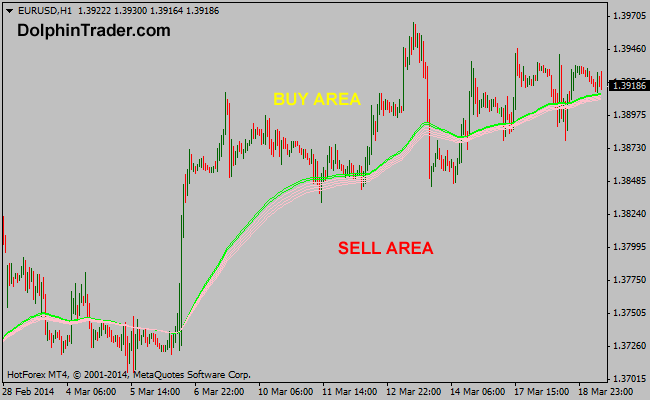# 71 EMA – 90 EMA Guppy Multi Moving Average Metatrader 4 Indicator

This is the fifth GMMA forex indicator in a series of 11 indicators entirely based on the Guppy multi moving average. The RainbowMMA_05 consists of the following moving averages: 90 period exponential moving average, 86 period exponential moving average, 82 period exponential moving average, 78 period exponential moving average, 74 period exponential moving average and 71 period exponential moving average.

Buy: Wait for the price to rise above the RainbowMMA_05 GMMA
Sell: Wait for the price to drop below the RainbowMMA_05 GMMA

Indicator Preferences

Currency pairs: any
Time frames: any
Sessions: any